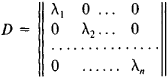# Symmetric Matrix

(redirected from Symmetric array)
Also found in: Dictionary.

## symmetric matrix

[sə′me·trik ′mā·triks]
(mathematics)
A matrix which equals its transpose.

## Symmetric Matrix

a square matrix S = ||sik|| in which any two elements that are symmetrically located with respect to the principal diagonal are equal: sik = ski, where i, k = 1, 2,…, n. A symmetric matrix is often treated as the matrix of the coefficients of some quadratic form. The theory of symmetric matrices and the theory of quadratic forms are closely related.

The properties of the spectrum of a symmetric matrix with real elements include the following: (1) all the roots λ1, λ2,…, λn of the characteristic equation of the matrix are real; and (2) to these roots there correspond n pairwise orthogonal eigenvectors of the matrix, where n is the order of the matrix. A symmetric matrix with real elements can always be represented in the form S″ = ODO-1, where O is an orthogonal matrix andSite: Follow: Share:
Open / Close